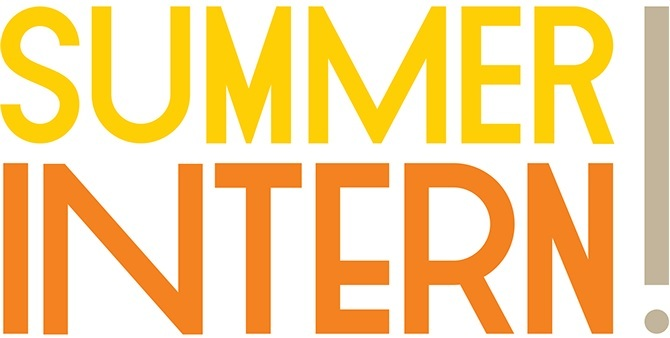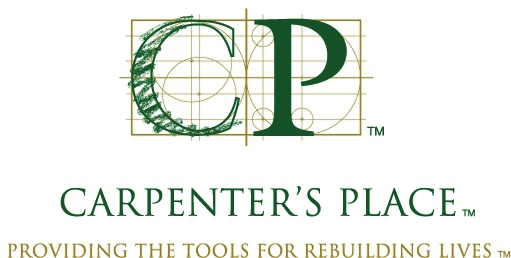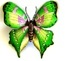table div table+table div table{width:100%;padding:0}table div table+table div table img{width:96.23%;padding:0;float:none}table div table+table div table td{width:100%;padding:0 1.88% 18px}/* styles */## This Week at St. Mark February 5-12, 2023

 table div table+table+table+table div table{width:100%;padding:0}table div table+table+table+table div table img{width:96.23%;padding:0;float:none}table div table+table+table+table div table td{width:100%;padding:0 1.88% 18px}/* styles */## Sunday, February 5

9:00 A.M. Worship with Live-stream
10:00 A.M. Fellowship Hour with coffee and donuts
10:10 A.M. Sunday School
11:00 A.M. Worship in the Sanctuary
6:30 P.M. Youth Group at St. Mark

## Monday, February 6

10:30 A.M. Classic Chorale Rehearses
2:00 P.M. St Mark Craft Group
5:30 P.M. Children's Ministry

## Tuesday, February 7

10:00 A.M. Staff Meeting
11:00 A.M. St. Mark Duplicate Bridge
1:00 P.M. First Tuesday Book Club at Peterson Meadows

## Wednesday, February 8

9:00 A.M. Women's Bible Study
9:00 A.M. Men's Bible Study
4:30 P.M. Outreach Ministry
6:30 P.M. Confirmation

## Thursday, February 9

9:00 A.M. Cherry Valley Bridge Group
9:30 A.M. Ruth Circle - at St. Mark
6:30 P.M. Emotions Anonymous
7:15 P.M. Red Lights

## Friday, February 10

7:30 A.M. St. Mark Breakfast Group at Denny's Restaurant

## Sunday, February 12 Annual Meeting

9:00 A.M. Worship with Live-Stream
10:10 A.M. Congregation Annual Meeting in the Sanctuary
6:30 P.M. Youth Event - Second Sunday at Grace Lutheran

 table div table+table+table+table+table+table div table{width:100%;padding:0}table div table+table+table+table+table+table div table img{width:96.23%;padding:0;float:none}table div table+table+table+table+table+table div table td{width:100%;padding:0 1.88% 18px}/* styles */## News and Events

 table div table+table+table+table+table+table+table+table div table{width:100%;padding:0}table div table+table+table+table+table+table+table+table div table img{width:96.23%;padding:0;float:none}table div table+table+table+table+table+table+table+table div table td{width:100%;padding:0 1.88% 18px}/* styles */table div table+table+table+table+table+table+table+table+table+table div table{width:100%;padding:0}table div table+table+table+table+table+table+table+table+table+table div table img{width:96.23%;padding:0;float:none}table div table+table+table+table+table+table+table+table+table+table div table td{width:100%;padding:0 1.88% 18px}/* styles */Sunday Morning Volunteers
Thank you to those of you who were able to be at the training last Sunday. We handed out guidelines for all volunteer duties and answered some good questions. There are copies of the guidelines and a contact sheet for each volunteer group. Please take one so if you need to find a replacement, you will have their email and phone numbers handy. If you have any questions, or concerns, please do not hesitate to contact the office, 815-398-3557.

 table div table+table+table+table+table+table+table+table+table+table+table+table div table{width:100%;padding:0}table div table+table+table+table+table+table+table+table+table+table+table+table div table img{width:96.23%;padding:0;float:none}table div table+table+table+table+table+table+table+table+table+table+table+table div table td{width:100%;padding:0 1.88% 18px}/* styles */Looking for a Summer Internship?
Attention College-aged members of St. Mark, the Summer Intern application for 2023 is live. Please click here at this link to fill out the application if you are interested in being the Summer Intern. If you have any questions please email Pastor
Katrina at prkatrina@stmarklc.com.

 table div table+table+table+table+table+table+table+table+table+table+table+table+table+table div table{width:100%;padding:0}table div table+table+table+table+table+table+table+table+table+table+table+table+table+table div table img{width:96.23%;padding:0;float:none}table div table+table+table+table+table+table+table+table+table+table+table+table+table+table div table td{width:100%;padding:0 1.88% 18px}/* styles */St Mark’s Annual Meeting
This year's annual congregation meeting is Sunday, February 12 at 10:10 a.m. It is very important as a member of St. Mark to take time to be here. There will be no 11:00 worship service this day, Sunday School or Adult Forum. The meeting will include the pastoral report on the state of the congregation, the election of officers and committee members, and a vote on amendments to our governing documents. The Fiscal 2023 budget will be presented for discussion and approval. All voting members are asked to participate. There will be plenty of fun activities for children in Fellowship Hall during the meeting.

Annual Reports Available
The 2022 Annual Congregation reports are available to pick up in the Narthex or stop by the church office during the week. Please only one per family.

 table div table+table+table+table+table+table+table+table+table+table+table+table+table+table+table+table div table{width:100%;padding:0}table div table+table+table+table+table+table+table+table+table+table+table+table+table+table+table+table div table img{width:96.23%;padding:0;float:none}table div table+table+table+table+table+table+table+table+table+table+table+table+table+table+table+table div table td{width:100%;padding:0 1.88% 18px}/* styles */St. Mark “Makers,” Craft Group
We plan to meet once a month and make a craft.
Join us Monday, February 6, 2:00-4:00 P.M. We will meet in the St. Mark cafe. The cost is \$5 to cover the cost of supplies. Bring your scissors. Text/call Betsy Ellenberger at 815-378-1429 to RSVP by February 2.

Save the Date for the next craft day, March 6.

 table div table+table+table+table+table+table+table+table+table+table+table+table+table+table+table+table+table+table div table{width:100%;padding:0}table div table+table+table+table+table+table+table+table+table+table+table+table+table+table+table+table+table+table div table img{width:96.23%;padding:0;float:none}table div table+table+table+table+table+table+table+table+table+table+table+table+table+table+table+table+table+table div table td{width:100%;padding:0 1.88% 18px}/* styles */Ruth Circle
Thursday, February 9 at 9:30 am in Fellowship Hall. Following the meeting, we will clean the kitchen and then have a salad potluck lunch. Please bring a salad to share.

 table div table+table+table+table+table+table+table+table+table+table+table+table+table+table+table+table+table+table+table+table div table{width:100%;padding:0}table div table+table+table+table+table+table+table+table+table+table+table+table+table+table+table+table+table+table+table+table div table img{width:96.23%;padding:0;float:none}table div table+table+table+table+table+table+table+table+table+table+table+table+table+table+table+table+table+table+table+table div table td{width:100%;padding:0 1.88% 18px}/* styles */First Tuesday Book Club
We will meet on February 7 at 1:00 P.M. at Peterson Meadows in the private dining room. Wilma Reinke will be hosting. Any and all are invited. Please let Wilma know if you are planning to attend. The book is "The Sentence" by Louise Erdrich and Betty Sparks will be reviewing it.

 table div table+table+table+table+table+table+table+table+table+table+table+table+table+table+table+table+table+table+table+table+table+table div table{width:100%;padding:0}table div table+table+table+table+table+table+table+table+table+table+table+table+table+table+table+table+table+table+table+table+table+table div table img{width:96.23%;padding:0;float:none}table div table+table+table+table+table+table+table+table+table+table+table+table+table+table+table+table+table+table+table+table+table+table div table td{width:100%;padding:0 1.88% 18px}/* styles */The 2023 Altar Flower Chart is Up
Each Sunday we have two beautiful bouquets of flowers on the altar. The 2023 flower chart is up in the narthex on the bulletin board. You may sign up on the chart, call the church office or click on this link. We will continue to purchase them from Cherry Blossom. The price per arrangement will stay the same \$40. If you sign up online, put your memory or honor of in the comment line and pay here or write a check to St. Mark. If you have the mobile app you can do all of it on there. You may take your flowers home after the service or stop by the office
during the week to pick them up.

 table div table+table+table+table+table+table+table+table+table+table+table+table+table+table+table+table+table+table+table+table+table+table+table+table div table{width:100%;padding:0}table div table+table+table+table+table+table+table+table+table+table+table+table+table+table+table+table+table+table+table+table+table+table+table+table div table img{width:96.23%;padding:0;float:none}table div table+table+table+table+table+table+table+table+table+table+table+table+table+table+table+table+table+table+table+table+table+table+table+table div table td{width:100%;padding:0 1.88% 18px}/* styles */## Outreach MinistryOutreach Opportunity at Carpenters Place
Here is a quick and easy opportunity for St. Mark members to help serve a noon lunch at Carpenters Place this year. NO FOOD PREPARATION - Just arrive at Carpenters Place by 11:45. We serve lunch from 12:00 til 12:30. No clean-up is involved. We need 3 or 4 volunteers for the following dates in 2023:

April 27, June 22, August 24, and October 26. Call or Text Keith @ 815-997-4499 if you are able to help.

 table div table+table+table+table+table+table+table+table+table+table+table+table+table+table+table+table+table+table+table+table+table+table+table+table+table+table+table div table{width:100%;padding:0}table div table+table+table+table+table+table+table+table+table+table+table+table+table+table+table+table+table+table+table+table+table+table+table+table+table+table+table div table img{width:96.23%;padding:0;float:none}table div table+table+table+table+table+table+table+table+table+table+table+table+table+table+table+table+table+table+table+table+table+table+table+table+table+table+table div table td{width:100%;padding:0 1.88% 18px}/* styles */## Youth MinistryFebruary Schedule
February 5 - St. Mark Youth Group
February 12 - Second Sunday at Grace Lutheran
February 19 - No Youth Group
February 26 - St. Mark Youth Group/* styles */
 table div table+table+table+table+table+table+table+table+table+table+table+table+table+table+table+table+table+table+table+table+table+table+table+table+table+table+table+table+table+table+table+table div table{width:100%;padding:0}table div table+table+table+table+table+table+table+table+table+table+table+table+table+table+table+table+table+table+table+table+table+table+table+table+table+table+table+table+table+table+table+table div table img{width:96.23%;padding:0;float:none}table div table+table+table+table+table+table+table+table+table+table+table+table+table+table+table+table+table+table+table+table+table+table+table+table+table+table+table+table+table+table+table+table div table td{width:100%;padding:0 1.88% 18px}/* styles */## Primetimers MinistryValentine's Day Lunch
Join us on Tuesday, February 14 about noon for lunch at Tavern on Clark to celebrate Valentine's Day. A day to celebrate our coming together once again, to enjoy our friendship, bring our memories to mind, laugh, and partake of good food and drink. Come ONE AND ALL
everyone is welcome to join us in our private room. Sam always takes good care of us and everyone will order off the menu. Sign up sheet will be available Sunday at the Primetimer's table.

 table div table+table+table+table+table+table+table+table+table+table+table+table+table+table+table+table+table+table+table+table+table+table+table+table+table+table+table+table+table+table+table+table+table+table+table div table{width:100%;padding:0}table div table+table+table+table+table+table+table+table+table+table+table+table+table+table+table+table+table+table+table+table+table+table+table+table+table+table+table+table+table+table+table+table+table+table+table div table img{width:96.23%;padding:0;float:none}table div table+table+table+table+table+table+table+table+table+table+table+table+table+table+table+table+table+table+table+table+table+table+table+table+table+table+table+table+table+table+table+table+table+table+table div table td{width:100%;padding:0 1.88% 18px}/* styles */table div table+table+table+table+table+table+table+table+table+table+table+table+table+table+table+table+table+table+table+table+table+table+table+table+table+table+table+table+table+table+table+table+table+table+table+table+table div table{width:100%;padding:0}table div table+table+table+table+table+table+table+table+table+table+table+table+table+table+table+table+table+table+table+table+table+table+table+table+table+table+table+table+table+table+table+table+table+table+table+table+table div table img{width:96.23%;padding:0;float:none}table div table+table+table+table+table+table+table+table+table+table+table+table+table+table+table+table+table+table+table+table+table+table+table+table+table+table+table+table+table+table+table+table+table+table+table+table+table div table td{width:100%;padding:0 1.88% 18px}/* styles */## Remember In Our Prayers

 table div table+table+table+table+table+table+table+table+table+table+table+table+table+table+table+table+table+table+table+table+table+table+table+table+table+table+table+table+table+table+table+table+table+table+table+table+table+table+table div table{width:100%;padding:0}table div table+table+table+table+table+table+table+table+table+table+table+table+table+table+table+table+table+table+table+table+table+table+table+table+table+table+table+table+table+table+table+table+table+table+table+table+table+table+table div table img{width:96.23%;padding:0;float:none}table div table+table+table+table+table+table+table+table+table+table+table+table+table+table+table+table+table+table+table+table+table+table+table+table+table+table+table+table+table+table+table+table+table+table+table+table+table+table+table div table td{width:100%;padding:0 1.88% 18px}/* styles */## Our St. Mark members:

Dewey Scheel, Lisa Belk, Lorine Kortus, Beth Johnson, Carol Kilts, Tim Panjkovich, Rita Olson, Fritzi Panjkovich, Bev Johnson, Jack Sowl, Jan Cotter, Diane Swanson, Sherri Lindquist, Bette Patterson.

## Family and friends of St. Mark members:

Denise Nichols, Carrie Nack, Larry Foster, Barbara, Buttchen, John Ayotte, Carrie Kielty, Ed Sobczyk, Jeff Basset, Elizabeth Woodworth, Carrie Nelson, Bill Hart, Ellie Krepfle, Don Minnis, Tom Montgomery, Jon Baylander, John Mortensen, Carolyn Neagle, Jenny Hobby, Bill King, Carolyn King, Norma Comstock.

## Those serving in the military and their families:

Logan Gehrke, Hunter Haggestad, Tristan Davenport, Scot Hornick,
Bailey Panjkovich, Jim Tammen, Brandon Ballenger, Taylor Eversole.

Strengthen and encourage those in public health services and in the medical profession: care-givers, nurses, attendants, doctors, all who commit themselves to care for the sick and their families.

 table div table+table+table+table+table+table+table+table+table+table+table+table+table+table+table+table+table+table+table+table+table+table+table+table+table+table+table+table+table+table+table+table+table+table+table+table+table+table+table+table+table div table{width:100%;padding:0}table div table+table+table+table+table+table+table+table+table+table+table+table+table+table+table+table+table+table+table+table+table+table+table+table+table+table+table+table+table+table+table+table+table+table+table+table+table+table+table+table+table div table img{width:96.23%;padding:0;float:none}table div table+table+table+table+table+table+table+table+table+table+table+table+table+table+table+table+table+table+table+table+table+table+table+table+table+table+table+table+table+table+table+table+table+table+table+table+table+table+table+table+table div table td{width:100%;padding:0 1.88% 18px}/* styles *//* styles */ Office Hours Monday through Thursday 9:00 A.M. - 4:00 P.M. Friday - 9:00 A.M. - 1:00 P.M. Telephone: 815-398-3557 Publications St. Mark Memos, the weekly e-newsletter, is distributed each Thursday. The deadline for submission is Monday by 3:00 P.M. Please click to send submissions. Worship Services Miss a Sunday at St. Mark? Please visit our website and watch the service online. Please click to watch.
 table div table+table+table+table+table+table+table+table+table+table+table+table+table+table+table+table+table+table+table+table+table+table+table+table+table+table+table+table+table+table+table+table+table+table+table+table+table+table+table+table+table+table+table div table{width:100%;padding:0}table div table+table+table+table+table+table+table+table+table+table+table+table+table+table+table+table+table+table+table+table+table+table+table+table+table+table+table+table+table+table+table+table+table+table+table+table+table+table+table+table+table+table+table div table img{width:96.23%;padding:0;float:none}table div table+table+table+table+table+table+table+table+table+table+table+table+table+table+table+table+table+table+table+table+table+table+table+table+table+table+table+table+table+table+table+table+table+table+table+table+table+table+table+table+table+table+table div table td{width:100%;padding:0 1.88% 18px}/* styles */